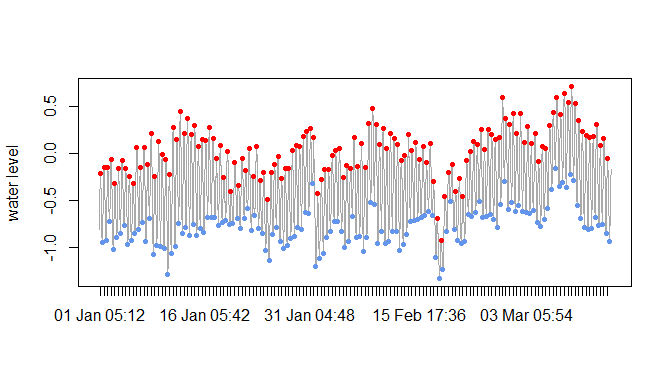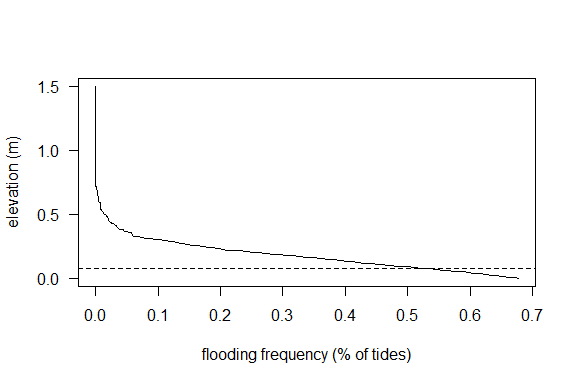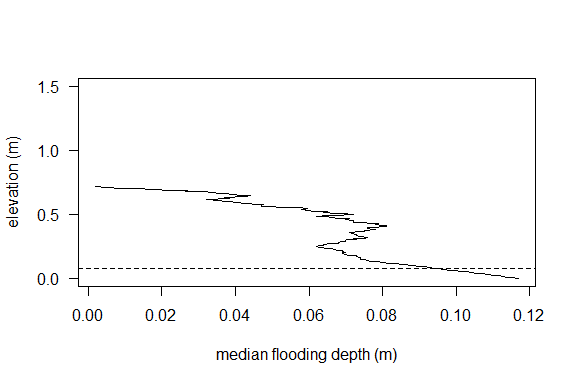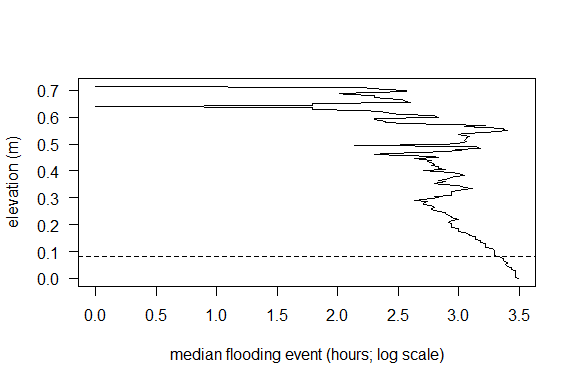# Analysis of tidal data

## Introduction

The VulnToolkit R package provides tools for summarizing and analyzing tidal data. It also includes interfaces to National Oceanic and Atmospheric Administration datasets, both time series data and station-level summary data for NOAA stations. This vignette deals with the former category, describing the functions useful in summary and analysis of tidal datasets.

## Data: New London water levels at 6 minute intervals

The NL_6min_2013 sample dataset included with VulnToolkit includes a year of tidal data for the New London NOAA station. The data were collected at 6-minute intervals and were downloaded using the VulnToolkit::noaa() function.

## Extracting useful information from tidal time series

### Tides

One of the basic functions we might want to perform on a tidal dataset is identification of high and low tides. This is accomplished using the HL() function and the results can be quickly visualized with the HL.plot function. If there are NAs present in the water level or time datasets used as inputs to HL and HL.plot, a console message (set using verbose = TRUE) can report the presence of NAS. The NAs are not removed or otherwise acted upon in the function; it is up to the user to decide on the proper treatment of NAs before or after identifying tides. Small periods of missing data may not be consequential, but could cause a high or low tide to be inaccurately characterized. By visualizing the raw data and the high/low tides identified by HL, the HL.plot function can be a useful tool for evaluating data quality.The HL and HL.plot functions take the same input arguments. HL.plot provides a simple means for the user to evaluate whether tides are being accurately identified, with high tides shown in red and low tides shown in blue, overlaid on the original water level time series. For long time series like the example above, it may be more informative to look closely at a subset of the data, to convince oneself that the high/low tides are correct.

The default arguments in both of these functions are aimed at capturing semidiurnal tides. For a dataset with diurnal tides, the period argument should be adjusted to reflect the longer tidal period (~26 hours).

Tides can be numbered using the number.tides function, helpful in quantifying material fluxes or in other analyses where tides are a unit of observation. This takes the high/low tides identified in the code above as an input argument.

nos <- number.tides(data = NL_6min_2013, datetime = NL_6min_2013[,1], hl = HL.NL)

### Flooding frequency and duration

Additional functions allow flooding regimes to be characterized based on the frequency of flooding and its duration. As one use case, this information can serve as the basis for evaluating how some change in sea level might affect flooding regimes.

# show how flooding frequency changes with elevation
elevs     <- data.frame(elev = seq(from = 0, to = 1.5, by = 0.005))
elevs$frq <- fld.frq(z = elevs$elev,
ht = HL.NL$level[HL.NL$tide == "H"])

plot(elev ~ frq, data = elevs, pch = 19, cex = 0.6, las = 1, type = "l",
xlab = "flooding frequency (% of tides)",
ylab = "elevation (m)")

# Let's look more closely at an elevation of interest: mean high water
MHW <- mean(HL.NL$level[HL.NL$tide == "H"])
abline(h = MHW, lty = 2) # add MHW line to plot# number of flooding tides at MHW
fld.frq(z = MHW, ht = HL.NL$level[HL.NL$tide == "H"], units = "tides") 
##  368
# flooding tides as a proportion of all tides in dataset
fld.frq(z = MHW, ht = HL.NL$level[HL.NL$tide == "H"], units = "percent")  
##  0.5219858

### Flooding depth

The depth of an average flooding event is another parameter describing a flooding regime. The fld.depth function reports the median depth from the observed flooding events along an elevation gradient.

elevs$median.depth <- fld.depth(elevation = elevs$elev,
level = NL_6min_2013[, 2])
plot(elevs$elev ~ elevs$median.depth ,
pch = 19, cex = 0.6, las = 1, type = "l",
xlab = "median flooding depth (m)",
ylab = "elevation (m)")
abline(h = MHW, lty = 2) # line indicates MHW### Duration of individual flooding events

The duration of an average flooding event can be an important metric for the flooding stress experienced by organisms occupying a wetland. This measure of the flooding regime also varies as a non-linear function of elevation:

elevs$events <- dur.events(elevation = elevs$elev,
level = NL_6min_2013[, 2])
plot(elevs$elev[!is.na(elevs$events)] ~
I(log(elevs$events[!is.na(elevs$events)])) ,
pch = 19, cex = 0.6, las = 1, type = "l",
xlab = "median flooding event (hours; log scale)",
ylab = "elevation (m)")
abline(h = MHW, lty = 2) # line indicates MHW